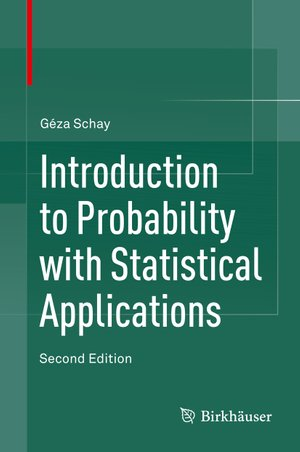Introduction to Probability with Statistical Applications

byGéza Schay

Pricing and Purchase Info

\$80.29 online
\$100.28 list price save 19%

Prices and offers may vary in store

Not available in stores

Now in its second edition, this textbook serves as an introduction to probability and statistics for non-mathematics majors who do not need the exhaustive detail and mathematical depth provided in more comprehensive treatments of the subject. The presentation covers the mathematical laws of random phenomena, including discrete and continuous random variables, expectation and variance, and common probability distributions such as the binomial, Poisson, and normal distributions. More classical examples such as Montmort's problem, the ballot problem, and Bertrand’s paradox are now included, along with applications such as the Maxwell-Boltzmann and Bose-Einstein distributions in physics.

Key features in new edition:

* 35 new exercises

* Expanded section on the algebra of sets

* Expanded chapters on probabilities to include more classical examples

* New section on regression

* Online instructors' manual containing solutions to all exercises<

Advanced undergraduate and graduate students in computer science, engineering, and other natural and social sciences with only a basic background in calculus will benefit from this introductory text balancing theory with applications.

Review of the first edition:

This textbook is a classical and well-written introduction to probability theory and statistics. … the book is written ‘for an audience such as computer science students, whose mathematical background is not very strong and who do not need the detail and mathematical depth of similar books written for mathematics or statistics majors.’ … Each new concept is clearly explained and is followed by many detailed examples. … numerous examples of calculations are given and proofs are well-detailed." (Sophie Lemaire, Mathematical Reviews, Issue 2008 m)

Title:Introduction to Probability with Statistical ApplicationsFormat:Kobo ebookPublished:June 17, 2016Publisher:Springer International PublishingLanguage:English

The following ISBNs are associated with this title:

ISBN - 10:3319306200

ISBN - 13:9783319306209

Appropriate for ages: All ages

Look for similar items by category: